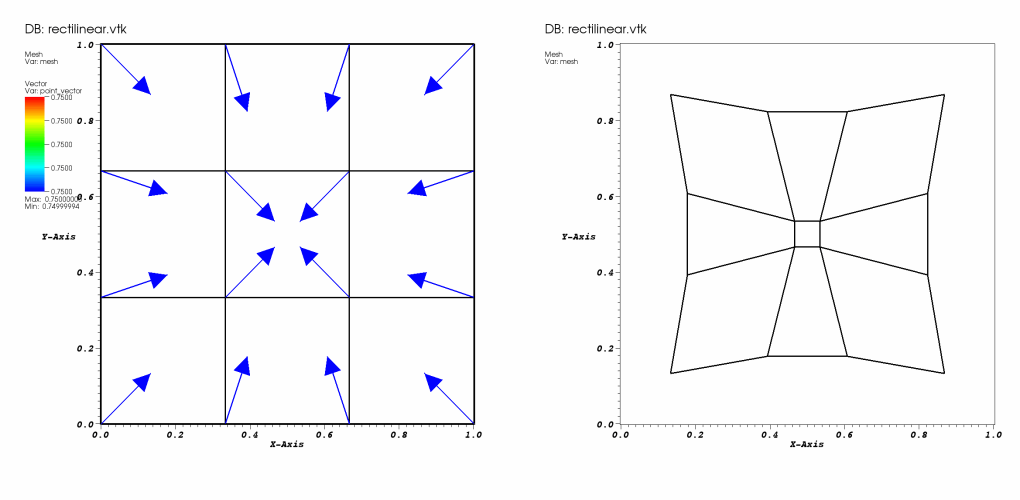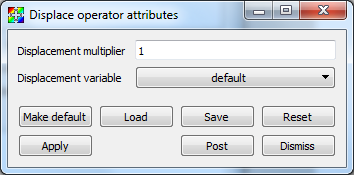# 4.3.9. Displace operator¶

The Displace operator deforms a mesh variable using a vector field that is defined on the nodes of that mesh. Many engineering simulation codes write a mesh for the first time state of the simulation and then write vector displacements for the mesh for subsequent time states. The Displace operator makes it possible to use the mesh and the time-varying vector field to observe the behavior of the mesh over time. The Displace operator provides a multiplier that can amplify the effects of the vector field on the mesh so slight changes in the vector field can be exaggerated. An example showing a mesh and a vector field, along with the results of the mesh displaced by the vector field is shown in Figure 4.30.Fig. 4.30 Mesh and Vector plots and a Mesh plot that uses the Displace operator to deform the mesh using a vector field.

## 4.3.9.1. Using the Displace operator¶

The Displace operator takes as inputs a mesh variable and a vector variable and a displacement multiplier value. For each node in the mesh, the Displace operator adds the vector field defined at that node to the node’s coordinates. Before adding the vector to the mesh, VisIt multiplies the vector by the displacement multiplier so the effects of the vector field can be exaggerated. To set a new value for the displacement multiplier, type a new value into the Displacement multiplier text field in the Displace attributes window (see Figure 4.31). To set the name of the vector variable that VisIt uses to displace the mesh, select a new vector variable from the Displacement variable variable button.Fig. 4.31 Displace attributes window